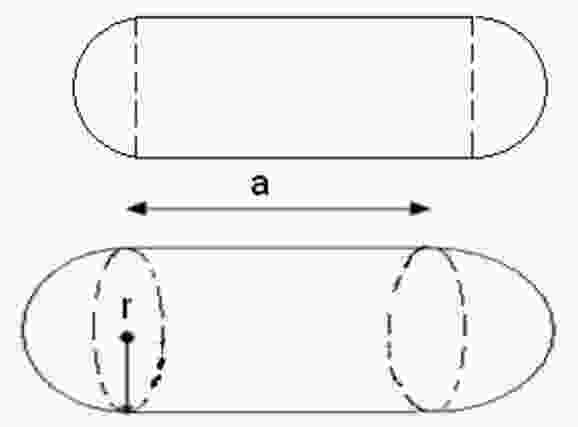### Volume of Cylinder with Hemispherical Ends Calculator

Calculate the volume of cylinder with Hemispherical ends for given Radius and length using the following formula:
Volume = (Pi X r2 X L) + (4 X Pi / 3 X r3)
for example: the capsule is a shape consisting of a cylinder with hemispherical ends means a sphere has been cut in half through its center and combined at both the ends of a cylinder.Radius Length Volume